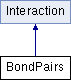ParM  parm A molecular dynamics library
BondPairs Class Reference

#include <interaction.hpp>

Inheritance diagram for BondPairs:## Public Member Functions

BondPairs (vector< BondGrouping > pairs, bool zeropressure=true)

BondPairs (bool zeropressure=true)

bool add (BondGrouping b, bool replace=true)
Add a pair of atoms. More...

bool add (flt k, flt x0, AtomID a1, AtomID a2, bool replace=true)

bool add (flt k, AtomID a1, AtomID a2, bool replace=true)
Add a pair of atoms with the current distance. More...

uint size () const

BondGrouping get (uint i) const

flt mean_dists (Box &box) const

flt std_dists (Box &box) const

flt energy (Box &box)
Potential energy due to this Interaction. More...

void set_forces (Box &box)

flt pressure (Box &box)
Partial pressure due to this Interaction. More...

flt set_forces_get_pressure (Box &box)
Set forces (Atom.f) and return $$P = \sum_{\left<i,j \right>} \vec r_{ij} \cdot \vec F_{ij}$$ at the same time (see pressure()). More...Public Member Functions inherited from Interaction
virtual Matrix stress (Box &box)
The force-moment tensor for the current simulation: More...

virtual ~Interaction ()

## Protected Attributes

bool zeropressure

vector< BondGroupingpairs

## Constructor & Destructor Documentation

 BondPairs::BondPairs ( vector< BondGrouping > pairs, bool zeropressure = true )
 BondPairs::BondPairs ( bool zeropressure = true )

## Member Function Documentation

 bool BondPairs::add ( BondGrouping b, bool replace = true )

If "replace", a previous pair found will be replaced by the new pair. If not "replace" and that pair of atoms is already inserted, an error will be thrown.

 bool BondPairs::add ( flt k, flt x0, AtomID a1, AtomID a2, bool replace = true )
inline
 bool BondPairs::add ( flt k, AtomID a1, AtomID a2, bool replace = true )
inline

Add a pair of atoms with the current distance.

 void BondPairs::add_forced ( BondGrouping b )
inline
 flt BondPairs::energy ( Box & box )
virtual

Potential energy due to this Interaction.

Implements Interaction.

 BondGrouping BondPairs::get ( uint i ) const
inline
 flt BondPairs::mean_dists ( Box & box ) const
 flt BondPairs::pressure ( Box & box )
virtual

Partial pressure due to this Interaction.

$$P = \sum_{\left<i,j \right>} \vec r_{ij} \cdot \vec F_{ij}$$, or equivalently $$P = \sum_i \vec r_i \cdot \vec F_i$$

Note that the full pressure involves all interactions and temperature, and needs to be normalized by $$\frac{1}{dV}$$ where $$d$$ is the number of dimensions and $$V$$ is the volume.

Implements Interaction.

 void BondPairs::set_forces ( Box & box )
virtual

Implements Interaction.

 flt BondPairs::set_forces_get_pressure ( Box & box )
virtual

Set forces (Atom.f) and return $$P = \sum_{\left<i,j \right>} \vec r_{ij} \cdot \vec F_{ij}$$ at the same time (see pressure()).

Reimplemented from Interaction.

 uint BondPairs::size ( ) const
inline
 flt BondPairs::std_dists ( Box & box ) const

## Member Data Documentation

 vector BondPairs::pairs
protected
 bool BondPairs::zeropressure
protected

The documentation for this class was generated from the following files: